Open in App
Not now

# Heap and Stack Memory Errors in Java

• Last Updated : 12 Sep, 2021

Memory allocation in java is managed by Java virtual machine in Java. It divides the memory into stack and heap memory which is as shown below in the below media as follows: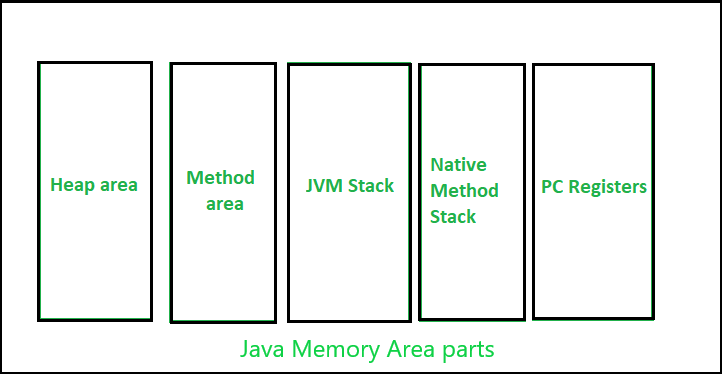Stack memory in Java

It is the temporary memory allocation where local variables, reference variables are allocated memory when their methods are called. It contains references to the object are stored in a heap. After the execution of the method, the memory containing those variables is cleared.  We can access this memory in Last In First Out Order. Allocation and deallocation is faster than heap memory. It is safer as data can only be accessed by the thread owner. If stack memory is full, then StackOverflowException is thrown by the JVM.

Illustration:

```// Java Program to Illustrate Stack Memory

// Importing required I/O classes
import java.io.*;

// Main class
class GFG {

// Main driver method
public static void main (String[] args) {

// Creating an integer array
int a [] = new int;
}
}```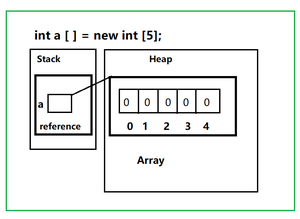Diagrammatic explanation of the above example

In the above illustration, we can conclusively perceive the above media shown and conclude out the following points

• ‘a’ is a variable of array type stored in a stack.
• new keyword is used to allocate memory in the heap.
• 5 is the size of the array.

Stack Memory Error

Whenever we call a method, after its execution it leaves the stack memory. If your methods are staying in the stack then the stack will be full, If the stack is full we can’t push, if we do then we will get the error java.lang.StackOverflowError which will be thrown by JVM. It is thrown when you call a method and there is no space left in the stack. In most cases, it is thrown when we are calling a method recursively without any proper termination condition. We can avoid it by making sure that methods are executing with proper termination.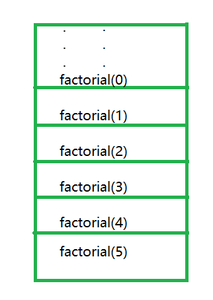After a certain point, stack will be full

Let us take a sample example of computing factorial of a number to illustrate the same.

## Java

 `// Java Program to Illustrate Stack Memory Error``// Factorial function without termination condition``// will cause StackOverflow error` `// Importing I/O classes``import` `java.io.*;` `// Main class``class` `GFG {` `    ``// Main driver method``    ``public` `static` `void` `main (String[] args) {` `        ``// Declaring a custom number whose factorial is to be computed``        ``int` `n = ``5``;` `        ``// Print and display the factorial``        ``System.out.println(factorial(n));``    ``}` `    ``// Method``    ``// To calculate factorial``    ``static` `int` `factorial(``int` `n) {` `        ``// Note: There is no termination condition` `        ``// Calling recursively to compute factorial of a number``        ``return` `n * factorial(n - ``1``);``    ``}``}`

Output: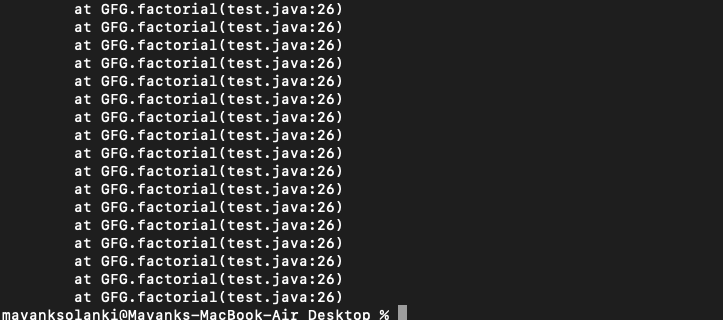Note: If you run this code you will get java.lang.StackOverflowError. We can avoid it by adding proper termination condition if we add termination condition in factorial function before return statement. Below termination condition remove error as follows:

if(n==0)

return 0;

Heap Memory in Java

Heap memory in java is used to allocate memory to the objects and JRE (Java Runtime Environment) classes. When an object is created, it is always created in heap and the reference to the object is stored in stack memory. It is not safe as a stack because it can be accessed globally. Access to this memory is relatively slower than the stack memory. It needs a garbage collector to remove unused objects. If the heap is full, java.lang.OutOfMemoryError is thrown by JVM. It is not thread-safe like a stack.

Example

## Java

 `// Java Program to Illustrate Execution in Heap Memory` `// Importing input output classes``import` `java.io.*;` `// Main class``class` `GFG {` `    ``// Static class``    ``static` `class` `Student {` `        ``int` `roll_no;` `        ``// The reference variable of String argument name``        ``// which points to the actual string from string``        ``// pool in heap memory``        ``String name;` `        ``// Constructor of this static class``        ``Student(``int` `roll_no, String name)``        ``{` `            ``// This keyword refers to current instance``            ``this``.roll_no = roll_no;``            ``this``.name = name;``        ``}``    ``}` `    ``// Main driver method``    ``public` `static` `void` `main(String[] args)``    ``{` `        ``// Primitive roll no value directly stored in stack``        ``// memory``        ``int` `roll_no = ``1``;``        ``// Primitive name value directly stored in stack``        ``// memory``        ``String name = ``"Jack"``;` `        ``// Creating reference variable of Student class type``        ``// created in a stack memory which will point to``        ``// the object in heap memory``        ``// New object created in heap memory``        ``Student st = ``new` `Student(roll_no, name);` `        ``// Print and display the student name and roll``        ``// number``        ``System.out.println(``"Student name -> "` `+ st.name);``        ``System.out.println(``"Student roll no. -> "``                           ``+ st.roll_no);``    ``}``}`

Output

```Student name -> Jack
Student roll no. -> 1```

Heap Memory Error

Also now it is suitable to discuss heap memory errors in java. So, it does occur when we creating lots of new objects in heap memory and there is no space left for new objects, then JVM will throw java.lang.OutOfMemoryError. Garbage collector removed the objects which have no references but cannot remove objects having a reference.  It can be avoided by removing references to unwanted objects.

Example:

## Java

 `// Java Program to Illustrate OutOfMemoryError``// in Heap Space` `// Importing input output classes``import` `java.io.*;` `// Main class``class` `GFG {` `    ``// Main driver method``    ``public` `static` `void` `main(String[] args)``    ``{` `        ``// Creating an array whose size is havoc``        ``Long a[] = ``new` `Long[``100000` `* ``10000``];``    ``}``}`

Output: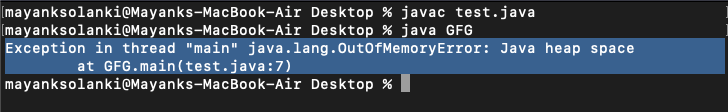Output explanation: In the above example, the Long array with a very large size is attempted to be initialized and the Java heap is insufficient to allocate this array, it throws a java.lang.OutOfMemoryError in java heap space.

My Personal Notes arrow_drop_up